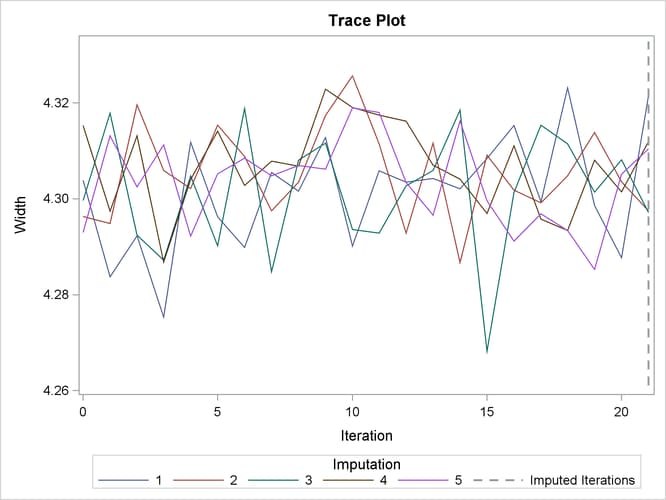# The MI Procedure

### Example 63.8 FCS Method with Trace Plot

This example uses FCS methods to impute missing values in both continuous and classification variables in a data set with an arbitrary missing pattern. The following statements use a logistic regression method to impute values of the classification variable `Species`:

```ods graphics on;
proc mi data=Fish3 seed=1305417 out=outex8;
class Species;
fcs plots=trace
logistic(Species= Length Width Length*Width /details link=glogit);
var Species Length Width;
run;
ods graphics off;
```

The "Model Information"  table in Output 63.8.1 describes the method and options used in the multiple imputation process. By default, a regression method is used to impute missing values in each continuous variable.

Output 63.8.1: Model Information

The MI Procedure

Model Information
Data Set WORK.FISH3
Method FCS
Number of Imputations 5
Number of Burn-in Iterations 20
Seed for random number generator 1305417

The "FCS Model Specification"  table in Output 63.8.2 describes methods and imputed variables in the imputation model. The procedure uses the logistic regression method to impute the variable `Species`, and the regression method to impute variables `Height` and `Width`.

Output 63.8.2: FCS Model Specification

FCS Model Specification
Method Imputed Variables
Regression Length Width
Logistic Regression Species

The "Missing Data Patterns" table in Output 63.8.3 lists distinct missing data patterns with corresponding frequencies and percentages.

Output 63.8.3: Missing Data Patterns

Missing Data Patterns
Group Species Length Width Freq Percent Group Means
Length Width
1 X X X 67 77.01 27.910448 4.361860
2 X X . 5 5.75 24.620000 .
3 X . X 6 6.90 . 4.167667
4 . X X 6 6.90 26.683333 4.136233
5 . X . 2 2.30 31.500000 .
6 . . X 1 1.15 . 3.663600

When you use the DETAILS keyword in the LOGISTIC option, parameters estimated from the observed data and the parameters used in each imputation are displayed in the "Logistic Models for FCS Method" table in Output 63.8.4.

Output 63.8.4: FCS Logistic Regression Model for `Species`

Logistic Models for FCS Method
Imputed
Variable
Effect Species Imputation
1 2 3 4 5
Species Intercept Parkki -2.172588 -2.324226 -2.418362 -1.832884 -0.929242
Species Intercept Perch 1.878263 0.445966 1.585375 0.919562 1.547549
Species Length Parkki 6.107448 6.377145 2.447654 -1.004869 2.363073
Species Length Perch -5.493897 -4.711566 -7.778194 -5.400749 -0.053788
Species Width Parkki -8.624156 -6.965179 -5.718729 -0.997851 -2.978868
Species Width Perch 8.111323 5.608314 9.426901 5.502755 1.241239
Species Length*Width Parkki -0.006404 2.138551 0.883903 0.072525 -0.152662
Species Length*Width Perch 1.151183 1.278025 1.117492 -0.195462 0.672738

With ODS Graphics enabled, the PLOTS=TRACE option displays trace plots of means for all continuous variables by default, as shown in Output 63.8.5 and Output 63.8.6. The dashed vertical lines indicate the imputed iterations—that is, the variable values used in the imputations. The plot shows no apparent trends for the two variables.

Output 63.8.5: Trace Plot for `Length`Output 63.8.6: Trace Plot for `Width`The following statements list the first 10 observations of the data set `Outex8` in Output 63.8.7:

```proc print data=outex8(obs=10);
title 'First 10 Observations of the Imputed Data Set';
run;
```

Output 63.8.7: Imputed Data Set

 First 10 Observations of the Imputed Data Set

Obs _Imputation_ Species Length Width
1 1 Roach 16.2000 2.26800
2 1 Roach 20.3000 2.82170
3 1 Roach 21.2000 3.40493
4 1 Roach 22.4203 3.17460
5 1 Roach 22.2000 3.57420
6 1 Roach 22.8000 3.35160
7 1 Roach 23.1000 3.39570
8 1 Roach 23.7000 3.73166
9 1 Roach 24.7000 3.75440
10 1 Roach 24.3000 3.54780

After the completion of five imputations by default, the "Variance Information" table in Output 63.8.8 displays the between-imputation variance, within-imputation variance, and total variance for combining complete-data inferences for continuous variables. The relative increase in variance due to missingness, the fraction of missing information, and the relative efficiency for each variable are also displayed. These statistics are described in the section Combining Inferences from Multiply Imputed Data Sets.

Output 63.8.8: Variance Information

Variance Information
Variable Variance DF Relative
Increase
in Variance
Fraction
Missing
Information
Relative
Efficiency
Between Within Total
Length 0.005177 0.815388 0.821601 83.332 0.007620 0.007590 0.998484
Width 0.000108 0.028944 0.029074 83.656 0.004496 0.004486 0.999104

The "Parameter Estimates" table in Output 63.8.9 displays a 95% mean confidence interval and a t statistic with its associated p-value for each of the hypotheses requested with the default MU0=0 option.

Output 63.8.9: Parameter Estimates

Parameter Estimates
Variable Mean Std Error 95% Confidence Limits DF Minimum Maximum Mu0 t for H0:
Mean=Mu0
Pr > |t|
Length 27.606967 0.906422 25.80424 29.40970 83.332 27.485512 27.675952 0 30.46 <.0001
Width 4.307702 0.170510 3.96860 4.64680 83.656 4.297146 4.321571 0 25.26 <.0001